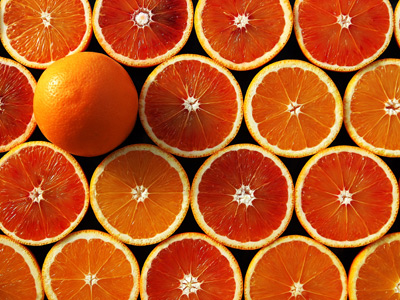Ben has x oranges and Jay has three more oranges than Ben.

# Algebra - Getting Started

This Math quiz is called 'Algebra - Getting Started' and it has been written by teachers to help you if you are studying the subject at middle school. Playing educational quizzes is a fabulous way to learn if you are in the 6th, 7th or 8th grade - aged 11 to 14.

It costs only \$12.50 per month to play this quiz and over 3,500 others that help you with your school work. You can subscribe on the page at Join Us

Algebra often substitutes letters for numbers. Where we could put a number we put a letter instead. Most advanced math relies on algebra so think of this quiz as a springboard into other exciting things!

Try your hand at Getting Started in Algebra.

1.
What is the meaning of 4x
4 divided by x
4 minus x
4 plus x
4 times x
Numbers and letters 'joined together' means multiply them together
2.
Sam has x guinea pigs, Paul has y guinea pigs and Joseph has z guinea pigs. We know that Sam and Paul together have the same number of guinea pigs as Joseph. How would we write this?
x + y = z
xyz
2x + y
x - y = z
3.
Jane has y candies and Lisa has two candies less than Jane. How would we write the number of candies that Lisa has?
2y
-2y
y - 2
y - y - y
4.
There are n boys in a football team. How would we write the number of boys in four football teams?
n x n x n x n
4 + n
n + 4
4n
The correct answer means the same as n + n + n + n
5.
Which of these is an 'expression'?
3x
3x + 4y
3x + 4y2
All of the above
An 'expression' contains one or more terms
6.
Thomas has x marbles and Richard has only one third as many. How would we write the number of marbles that Richard has?
x + 3
x - 3
x / 3
3x
7.
Which of these is a single 'term'?
2a
2a + b2
3a + 2b
3a + b + c
2a is a 'term', the other answers contain more than one 'term'
8.
Which of these is not an 'equation'?
3x = 7
4x2 - 6 = 28
4x + 5y + 6z
5x = 6y
An 'equation' always contains an = sign
9.
Peter has x oranges and Joe has three more oranges than Peter. How would we write the number of oranges that Joe has?
3x
x + 3
x + x + x + x
x3
10.
One of the following would never be written - which one?
x + y
1x + y
3x + 2y
4x - 6y